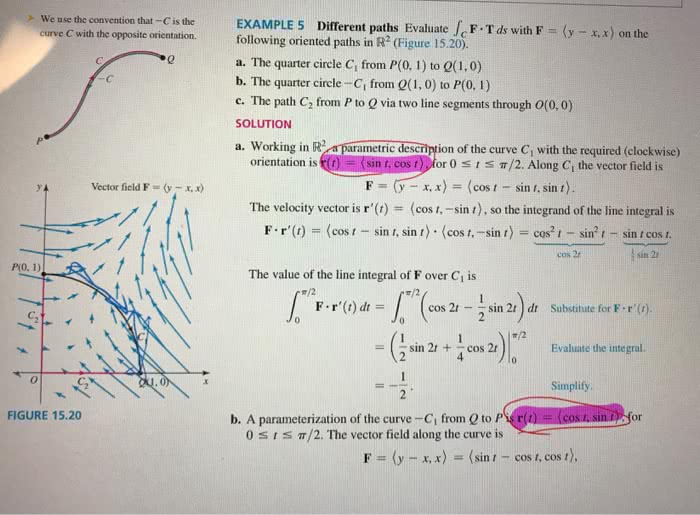1
0
watching
110
views13 Nov 2019

#Donât understand why the r(t) in part(a) is <sint, cost> and in part(b) is <cost, sint>. Thank you. Cis the EXAMPLE 5 Dirferent paths Evaluate F.Tds with F (y-.x) on the e C with the oppositie orientation.following oriented paths in R (Figure 15.20) a. The quarter circle C, from P(0, 1) to Q(1.0) b. The quarter circle -C, from Q(1, 0) to P(O. 1) c. The path C2 from P to Q via two line segments through 0(0,0) SOLUTION a. Working in R metric descripion of the curve C, with the required (clockwise) orientation is or 0 1 Ï/2. Along Cl the vector field is in F-(y-x, x)-(cost-sint, sint). The velocity vector is r'(t) (cos sin t), so the integrand of the line integral is F . r' (t)-( cos 1 _ sin r, sin r) . ãcos t,ã¼sin t-cos,-sin,-sin t cos t. y A vector field F = (y-x,x) cos 2t sin 2t The value of the line integral of F over Ci is F-r'(t) dt= / (cos 2t--sin 2t)dt Substitute for F . r'(t) Ï/2 -(isin 2, + Äcos 2)ã«a Evaluate the integral. Simplify FIGURE 15.20 b. A parameterization of the curve-Ci from Q to Pigr 0 1 Ï/2. The vector field along the curve is or F-(y-x,x)-(sin ,-cos ,, cos ,),